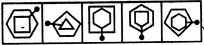# Non Verbal Reasoning - Classification - Discussion

### Discussion :: Classification - Section 1 (Q.No.49)

In each problem, out of the five figures marked (1), (2), (3), (4) and (5), four are similar in a certain manner. However, one figure is not like the other four. Choose the figure which is different from the rest.

49.

Choose the figure which is different from the rest.(1)     (2)     (3)     (4)     (5)

 [A]. 1 [B]. 2 [C]. 3 [D]. 4 [E]. 5

Explanation:

Only in fig. (4), the pin passes through a vertex of each one of the two elements.

 Anshuman said: (Dec 25, 2010) Only in fig. (3), the pin is not passes through a vertex of each one of the five elements.

 Nagendra said: (Jun 27, 2011) I think ans is 2 because all figure have hexagon shape except fig (2). This is major difference than pin passes through the vertex.

 Avinash Sonawane said: (Mar 8, 2017) Only in figure, pin is passing through both corners of figures. And in rest it passing through one corner and one side.

 Manikant Sharma said: (Jul 4, 2017) The answer should be option E. Because this is the only figure in which the internal figure touching the external figure.

 Monique said: (Nov 12, 2019) I think figure 1 because it is the only one that has 2 geometrical figures, the line segment, and a dot. No other figure has a dot.